# RD Sharma Solutions for Class 10 Maths Chapter 3 Pair of Linear Equations In Two Variables Exercise 3.4

The method of cross-multiplication is widely discussed in this exercise. Solving the systems of equations by the method of cross-multiplication is clearly explained in the RD Sharma Solutions Class 10. It’s a valuable resource developed by experts at BYJU’S for the purpose of clarifying doubts and help students focus on their weaker areas. Further, students can also make use of the RD Sharma Solutions for Class 10 Maths Chapter 3 Pair Of Linear Equations In Two Variables Exercise 3.4 PDF given below.

## RD Sharma Solutions for Class 10 Maths Chapter 3 Pair of Linear Equations In Two Variables Exercise 3.4 Download PDF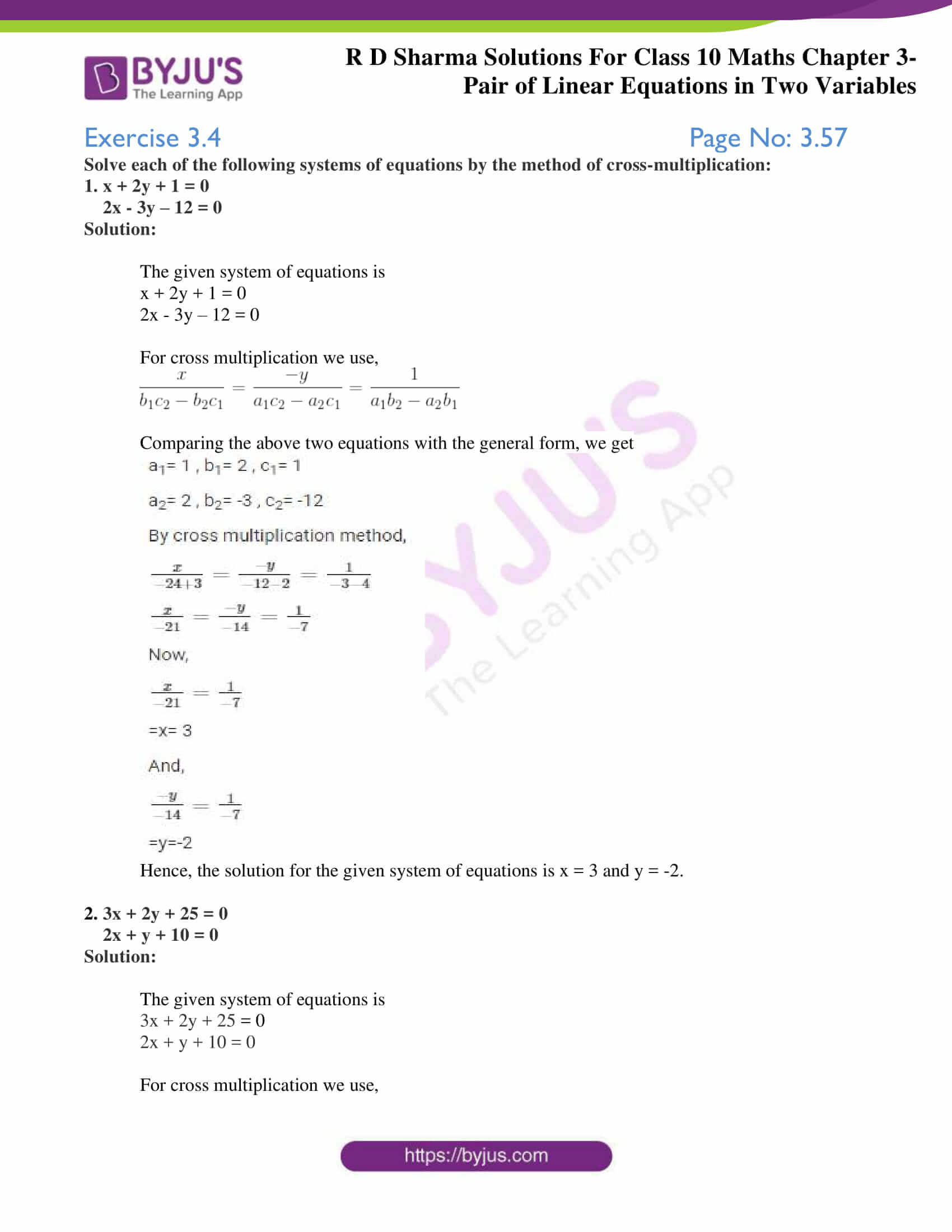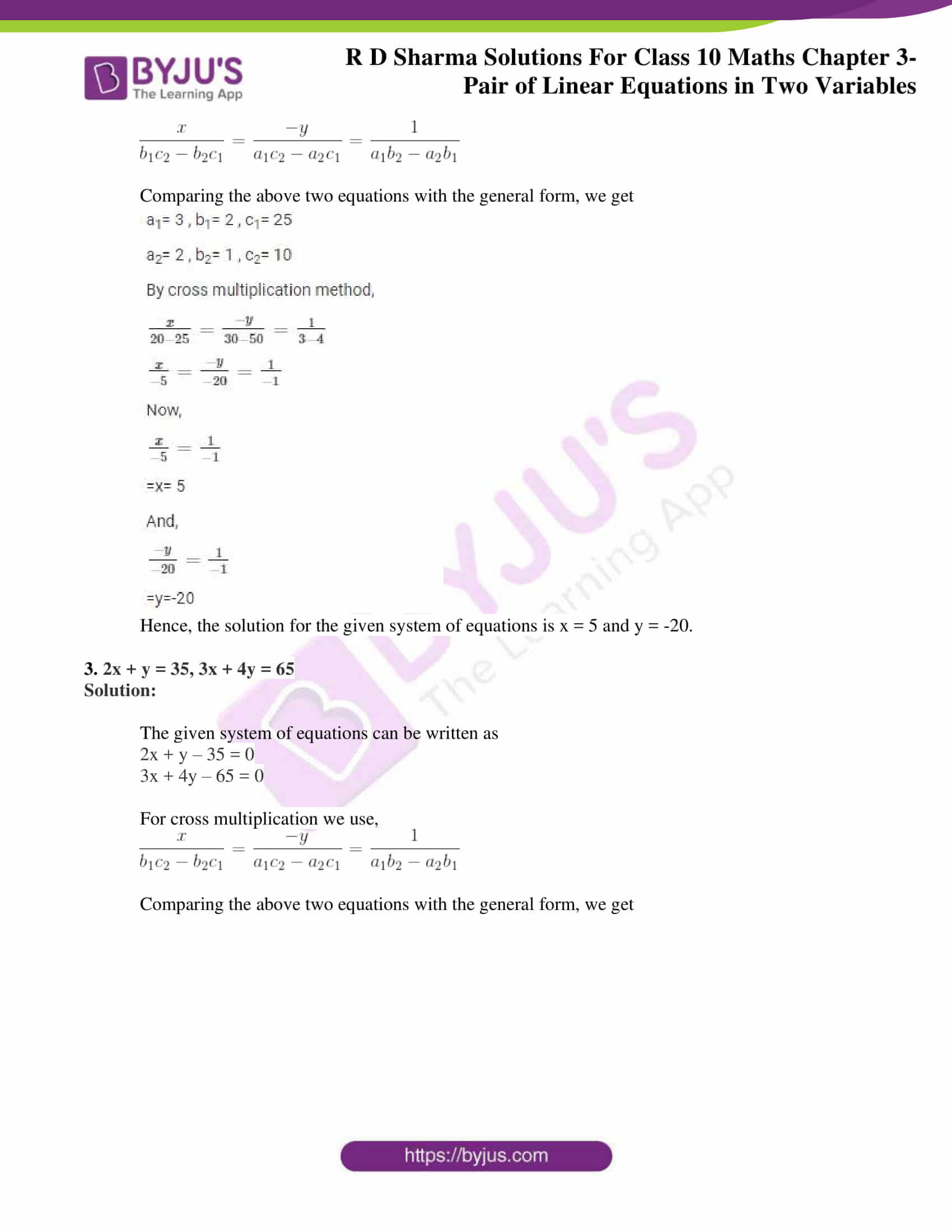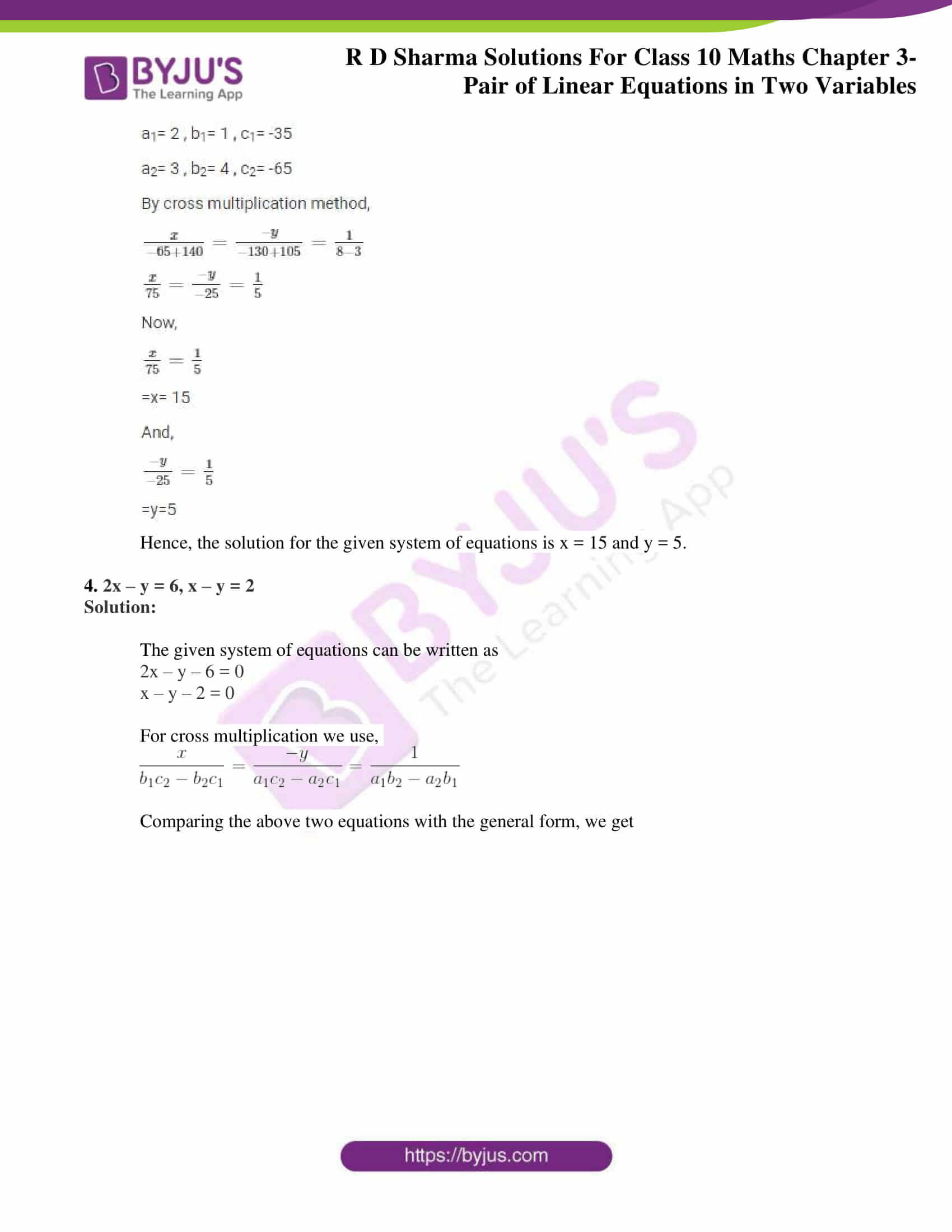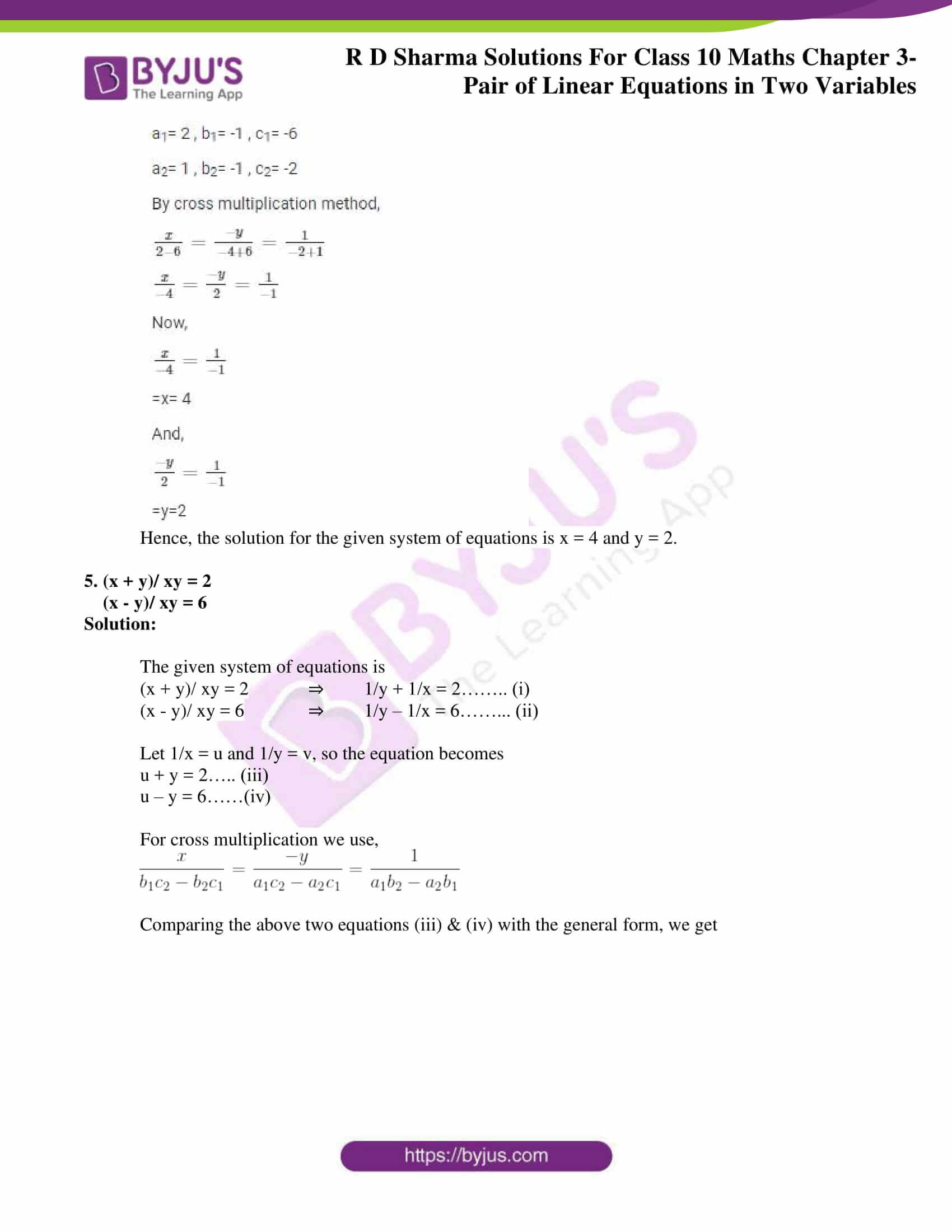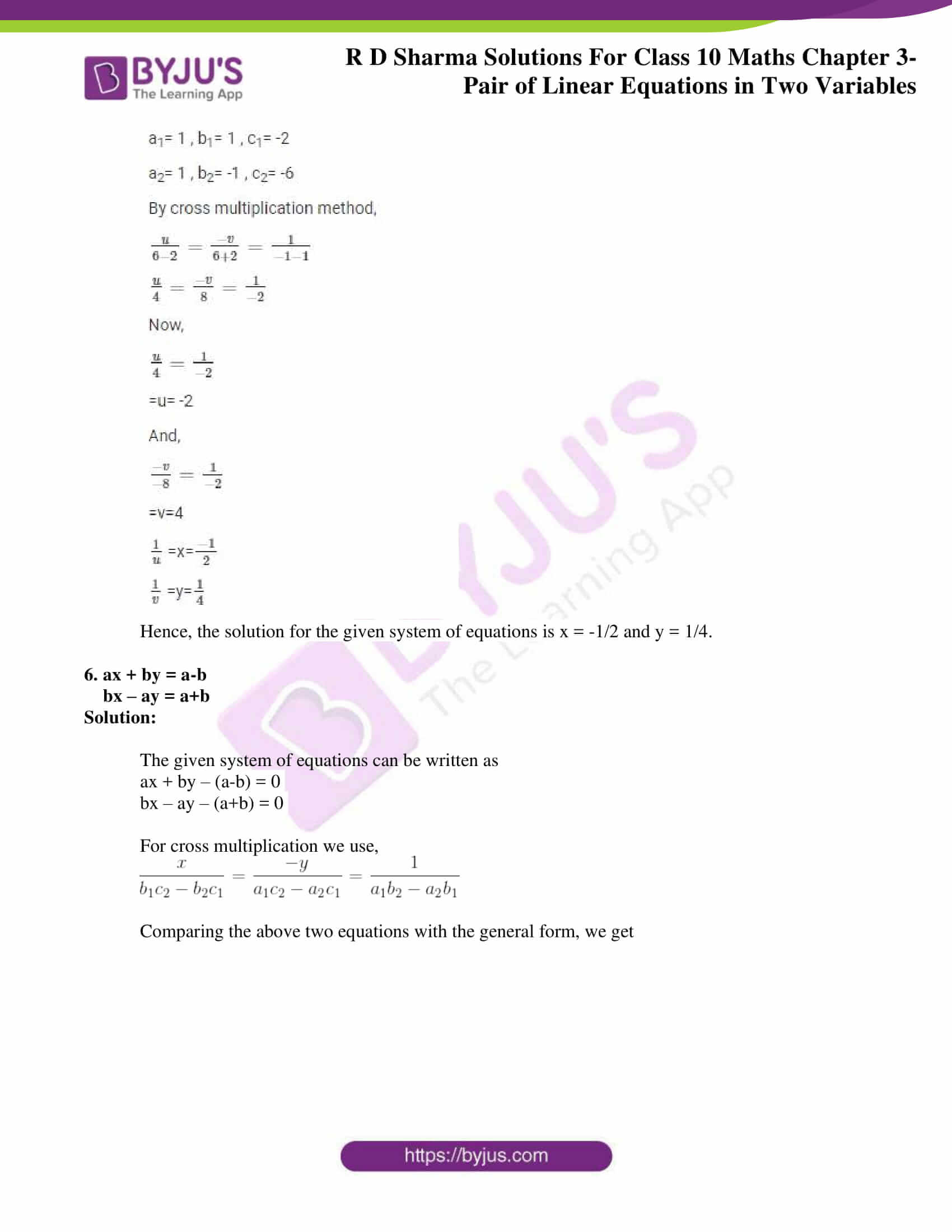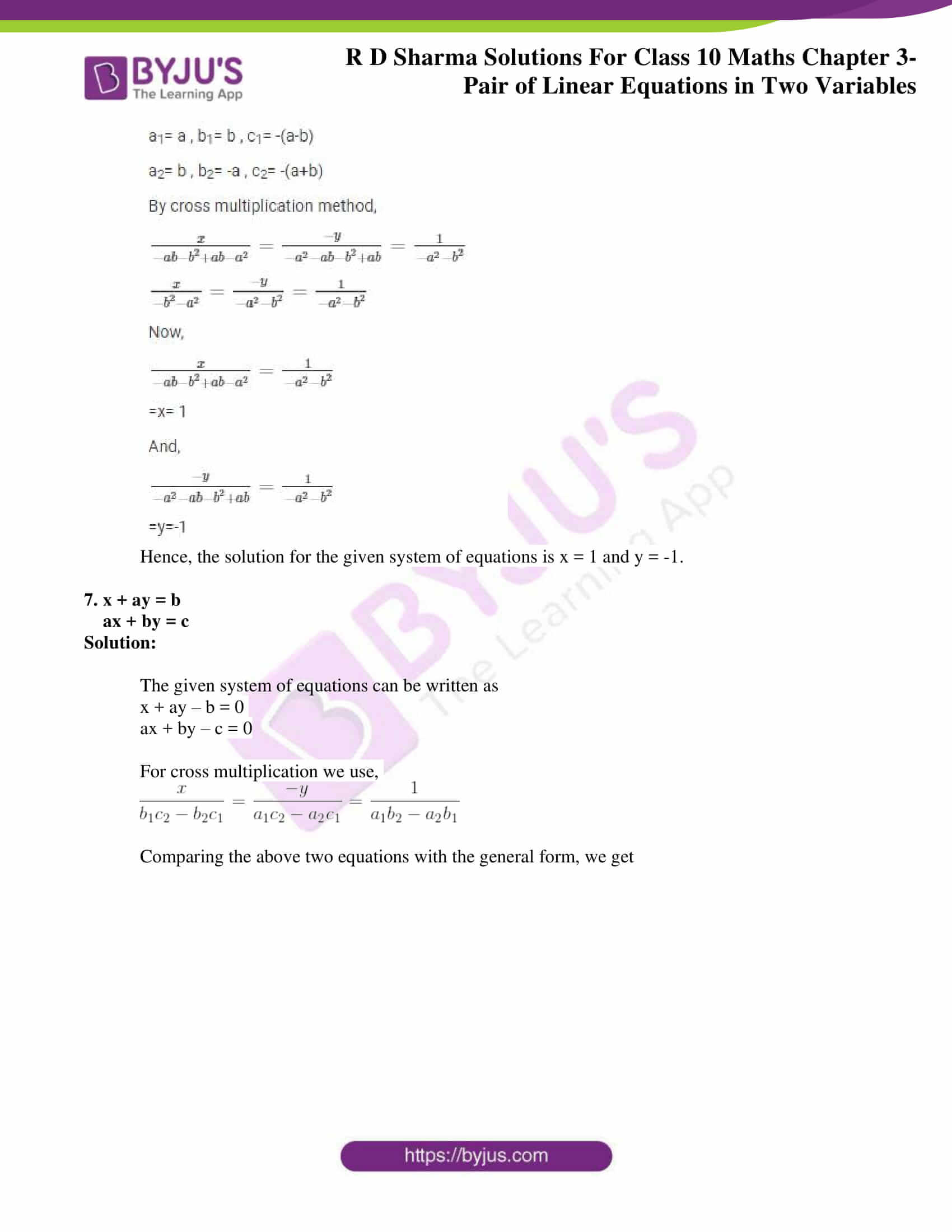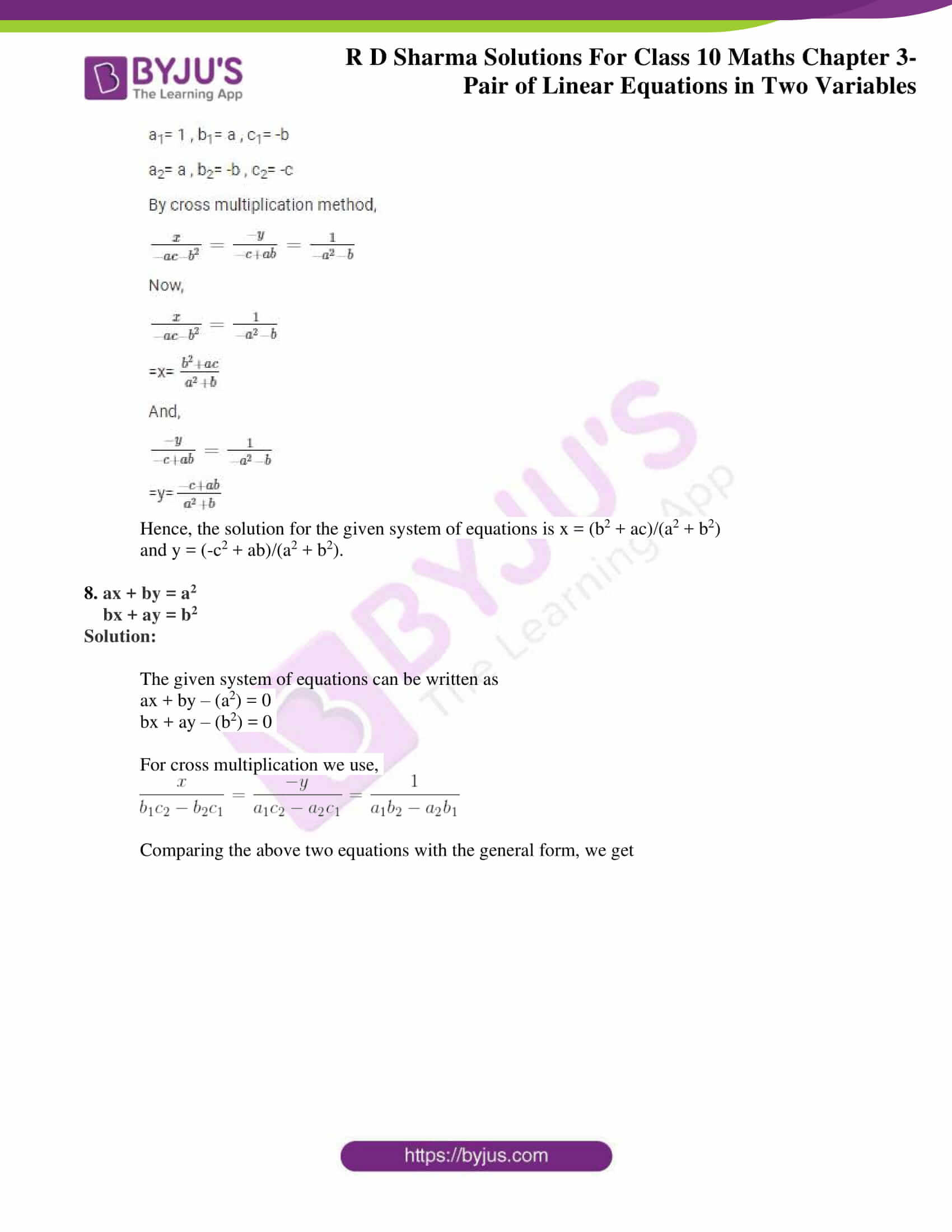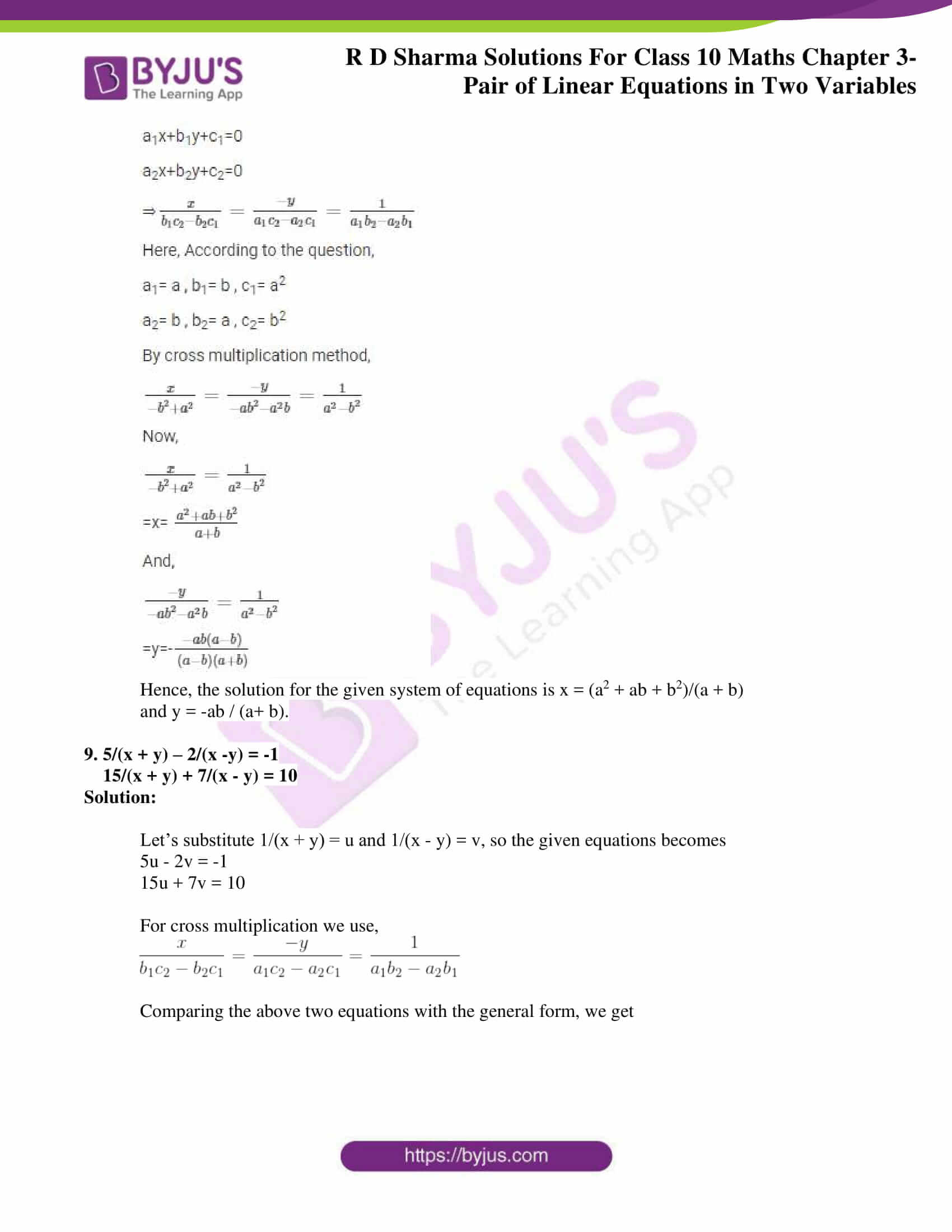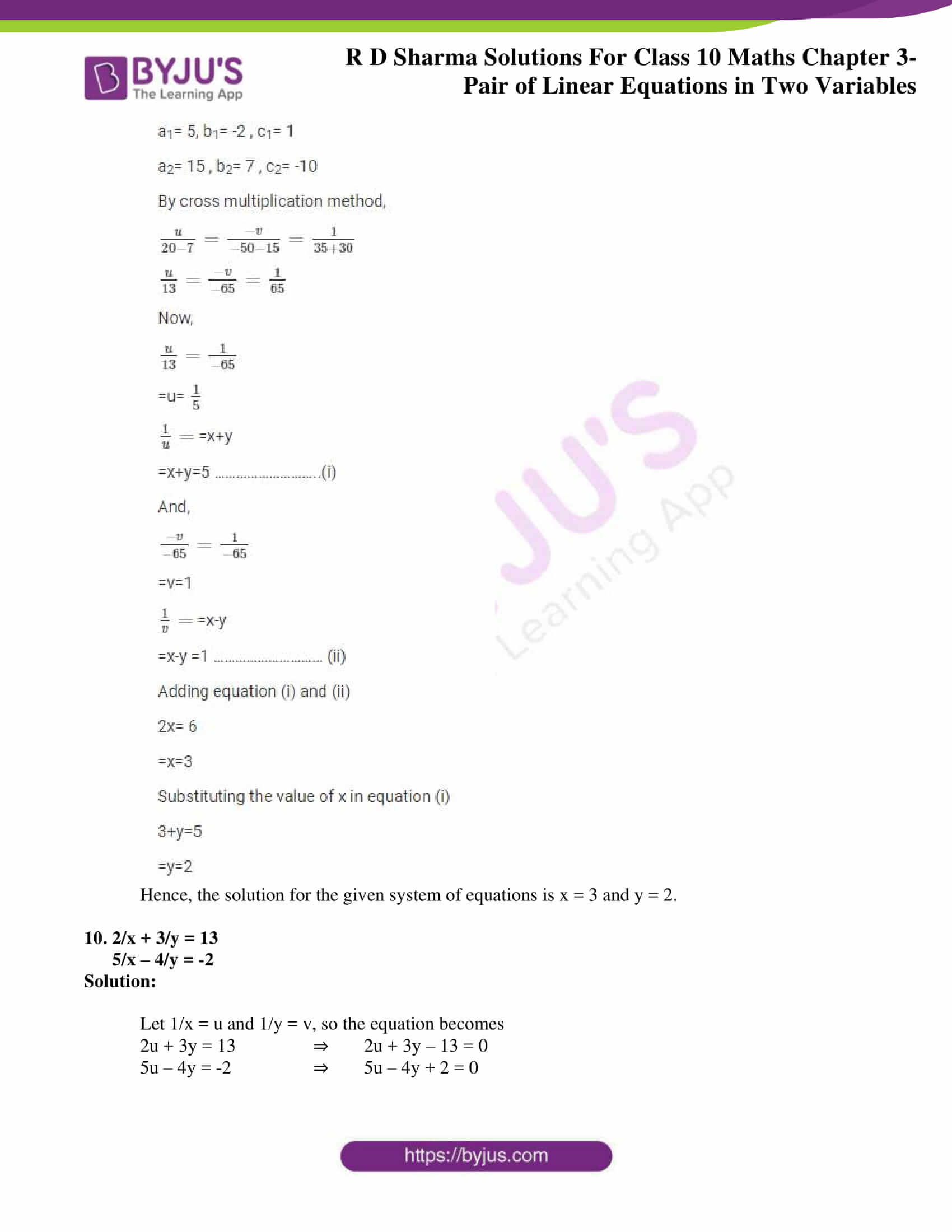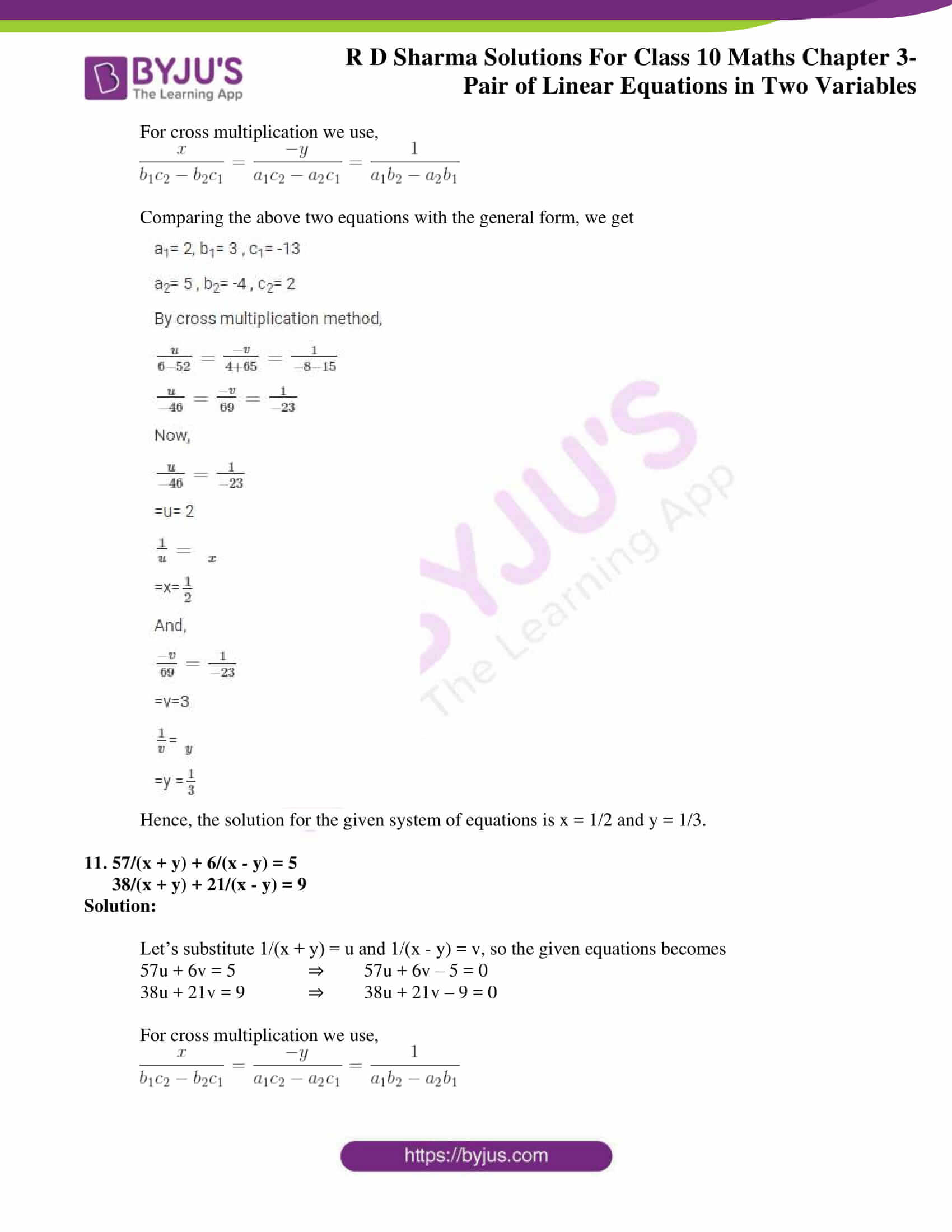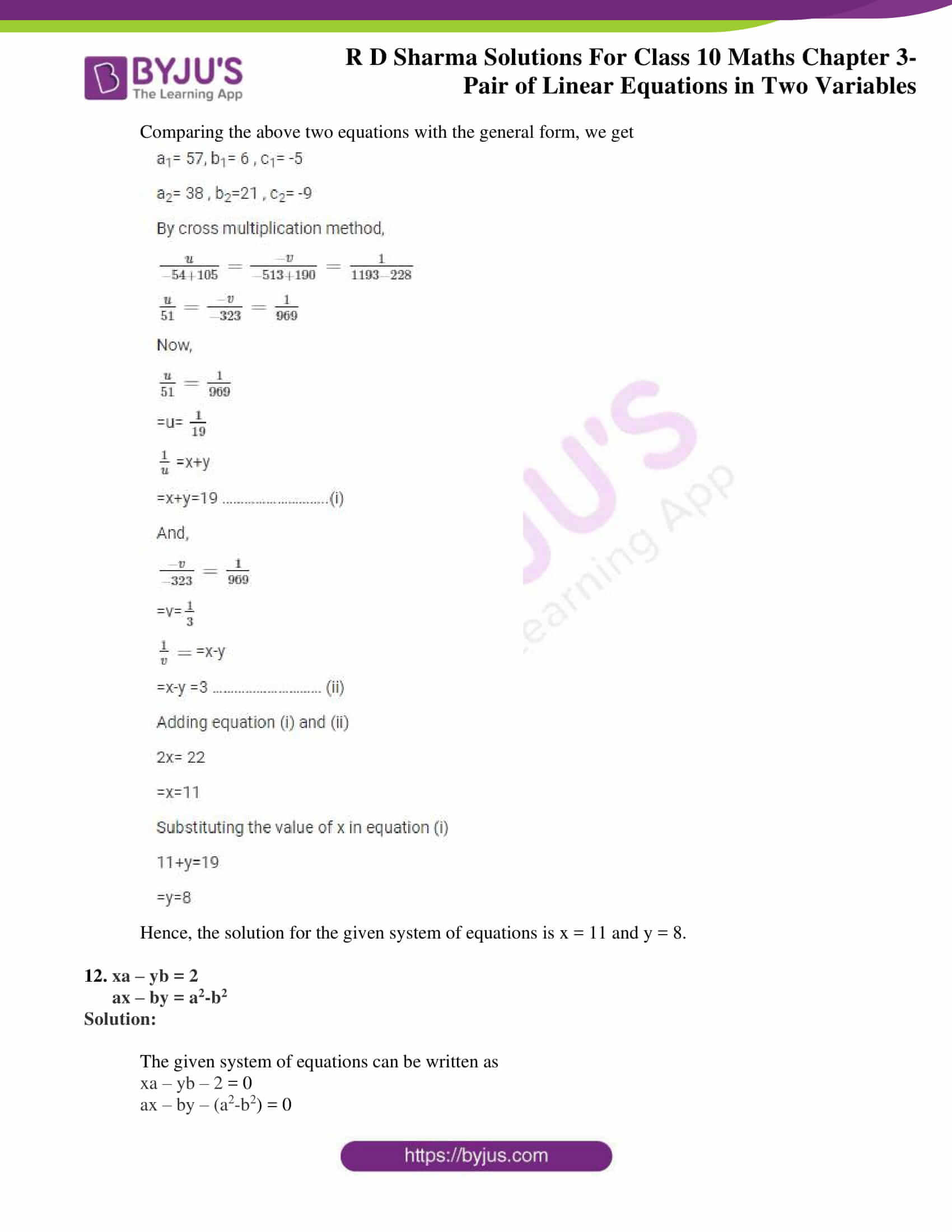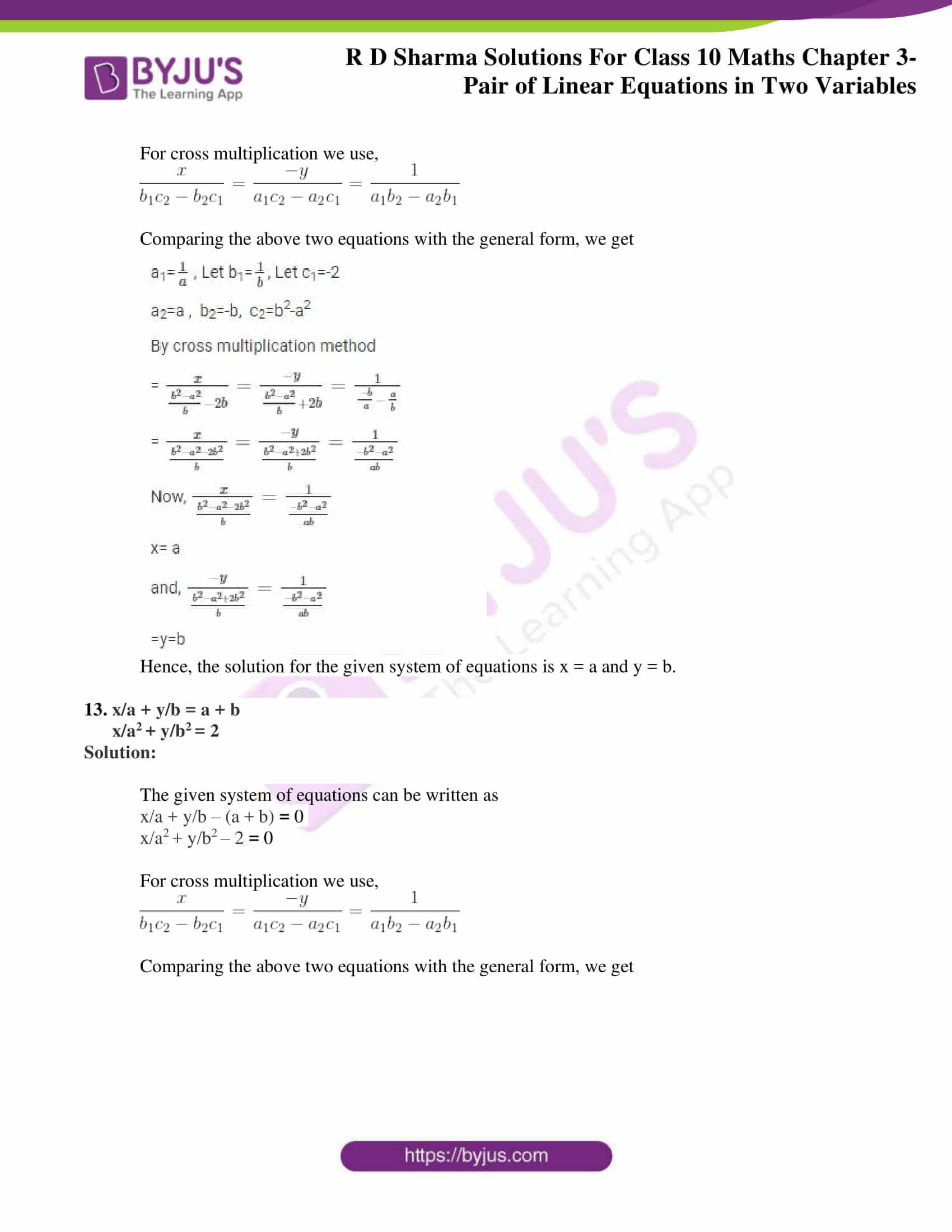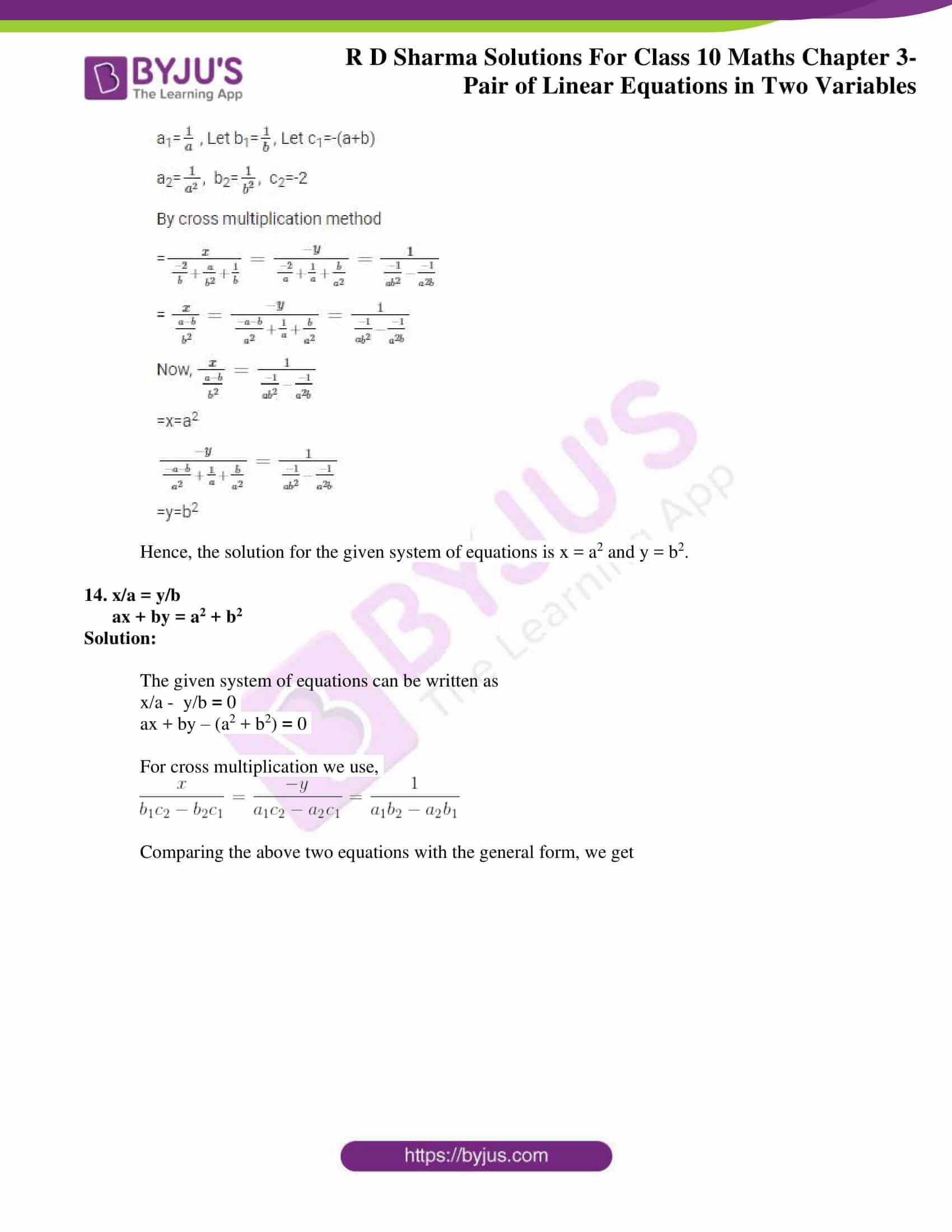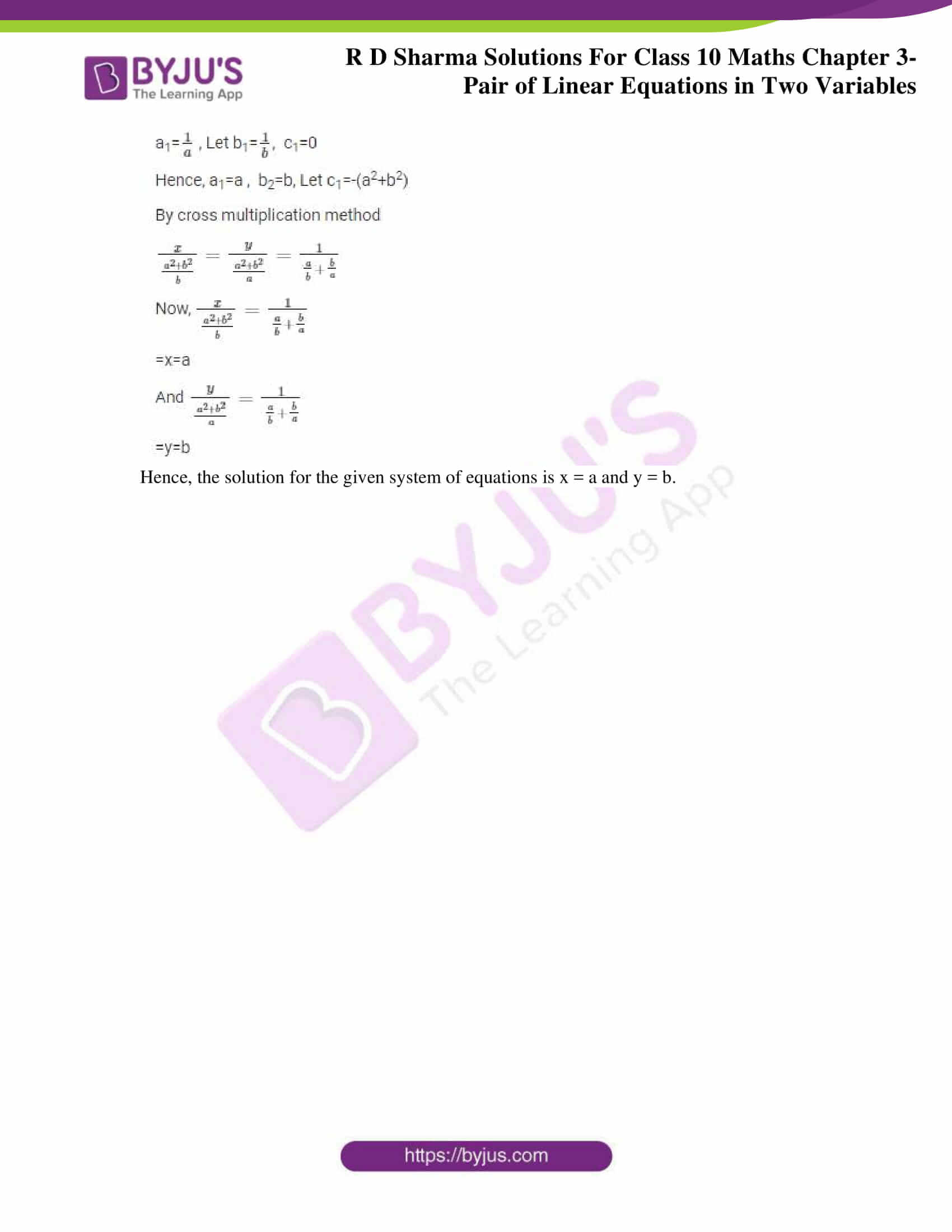### Access RD Sharma Solutions for Class 10 Maths Chapter 3 Pair of Linear Equations In Two Variables Exercise 3.4

Solve each of the following systems of equations by the method of cross-multiplication:

1. x + 2y + 1 = 0

2x – 3y – 12 = 0

Solution:

The given system of equations is

x + 2y + 1 = 0

2x – 3y – 12 = 0

For cross multiplication we use,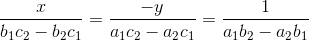Comparing the above two equations with the general form, we get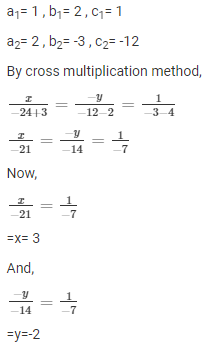Hence, the solution for the given system of equations is x = 3 and y = -2.

2. 3x + 2y + 25 = 0

2x + y + 10 = 0

Solution:

The given system of equations is

3x + 2y + 25 = 0

2x + y + 10 = 0

For cross multiplication we use,Comparing the above two equations with the general form, we get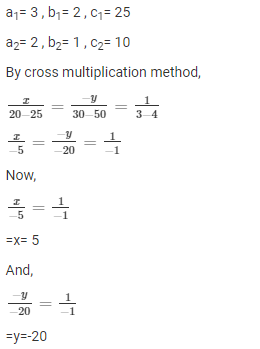Hence, the solution for the given system of equations is x = 5 and y = -20.

3. 2x + y = 35, 3x + 4y = 65

Solution:

The given system of equations can be written as

2x + y – 35 = 0

3x + 4y – 65 = 0

For cross multiplication we use,Comparing the above two equations with the general form, we get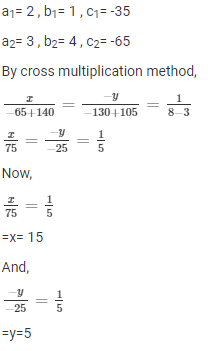Hence, the solution for the given system of equations is x = 15 and y = 5.

4. 2x – y = 6, x – y = 2

Solution:

The given system of equations can be written as

2x – y – 6 = 0

x – y – 2 = 0

For cross multiplication we use,Comparing the above two equations with the general form, we get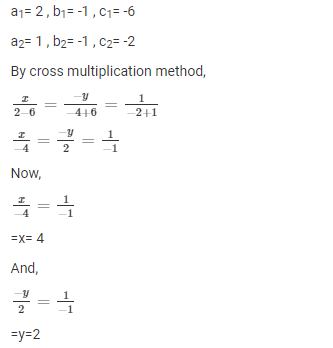Hence, the solution for the given system of equations is x = 4 and y = 2.

5. (x + y)/ xy = 2

(x – y)/ xy = 6

Solution:

The given system of equations is

(x + y)/ xy = 2 ⇒ 1/y + 1/x = 2…….. (i)

(x – y)/ xy = 6 ⇒ 1/y – 1/x = 6……… (ii)

Let 1/x = u and 1/y = v, so the equation becomes

u + y = 2….. (iii)

u – y = 6……(iv)

For cross multiplication we use,Comparing the above two equations (iii) & (iv) with the general form, we get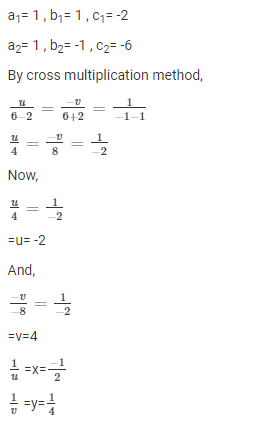Hence, the solution for the given system of equations is x = -1/2 and y = 1/4.

6. ax + by = a-b

bx – ay = a+b

Solution:

The given system of equations can be written as

ax + by – (a-b) = 0

bx – ay – (a+b) = 0

For cross multiplication we use,Comparing the above two equations with the general form, we get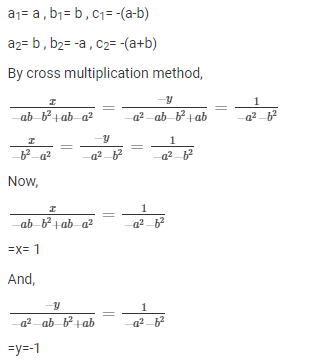Hence, the solution for the given system of equations is x = 1 and y = -1.

7. x + ay = b

ax + by = c

Solution:

The given system of equations can be written as

x + ay – b = 0

ax + by – c = 0

For cross multiplication we use,Comparing the above two equations with the general form, we get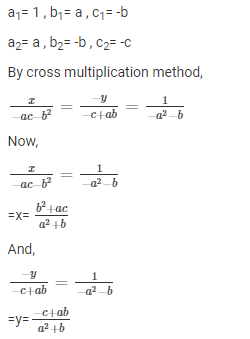Hence, the solution for the given system of equations is x = (b2 + ac)/(a2 + b2)

and y = (-c2 + ab)/(a2 + b2).

8. ax + by = a2

bx + ay = b2

Solution:

The given system of equations can be written as

ax + by – (a2) = 0

bx + ay – (b2) = 0

For cross multiplication we use,Comparing the above two equations with the general form, we get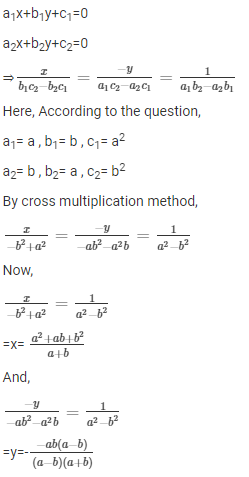Hence, the solution for the given system of equations is x = (a2 + ab + b2)/(a + b)

and y = -ab / (a+ b).

9. 5/(x + y) – 2/(x -y) = -1

15/(x + y) + 7/(x – y) = 10

Solution:

Let’s substitute 1/(x + y) = u and 1/(x – y) = v, so the given equations becomes

5u – 2v = -1

15u + 7v = 10

For cross multiplication we use,Comparing the above two equations with the general form, we get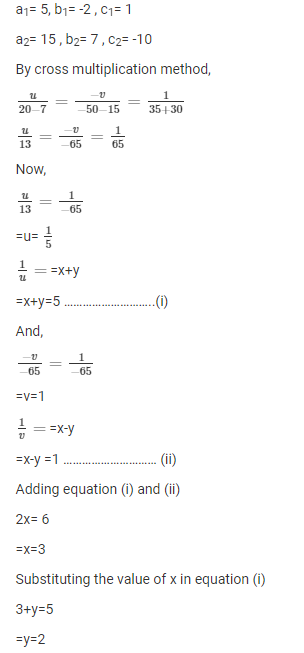Hence, the solution for the given system of equations is x = 3 and y = 2.

10. 2/x + 3/y = 13

5/x – 4/y = -2

Solution:

Let 1/x = u and 1/y = v, so the equation becomes

2u + 3y = 13 ⇒ 2u + 3y – 13 = 0

5u – 4y = -2 ⇒ 5u – 4y + 2 = 0

For cross multiplication we use,Comparing the above two equations with the general form, we get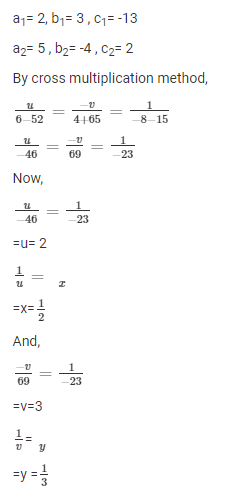Hence, the solution for the given system of equations is x = 1/2 and y = 1/3.

11. 57/(x + y) + 6/(x – y) = 5

38/(x + y) + 21/(x – y) = 9

Solution:

Let’s substitute 1/(x + y) = u and 1/(x – y) = v, so the given equations becomes

57u + 6v = 5 ⇒ 57u + 6v – 5 = 0

38u + 21v = 9 ⇒ 38u + 21v – 9 = 0

For cross multiplication we use,Comparing the above two equations with the general form, we get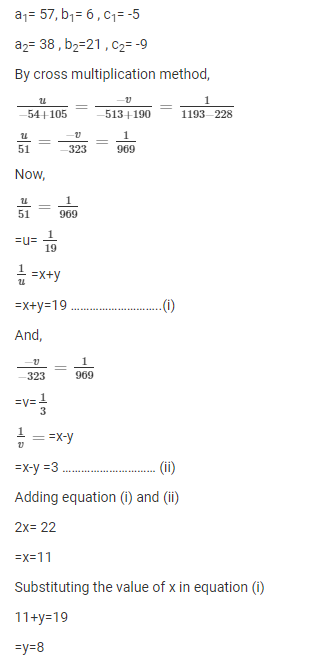Hence, the solution for the given system of equations is x = 11 and y = 8.

12. xa – yb = 2

ax – by = a2-b2

Solution:

The given system of equations can be written as

xa – yb – 2 = 0

ax – by – (a2-b2) = 0

For cross multiplication we use,Comparing the above two equations with the general form, we get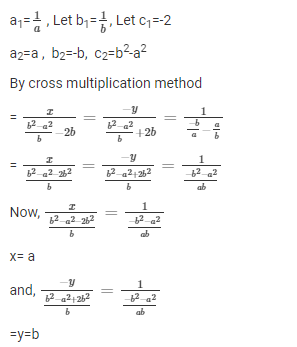Hence, the solution for the given system of equations is x = a and y = b.

13. x/a + y/b = a + b

x/a2 + y/b2 = 2

Solution:

The given system of equations can be written as

x/a + y/b – (a + b) = 0

x/a2 + y/b2 – 2 = 0

For cross multiplication we use,Comparing the above two equations with the general form, we get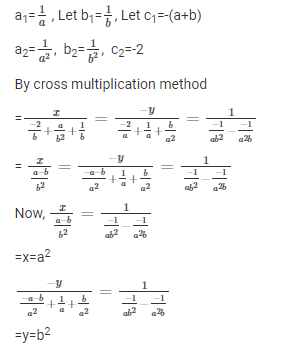Hence, the solution for the given system of equations is x = a2 and y = b2.

14. x/a = y/b

ax + by = a2 + b2

Solution:

The given system of equations can be written as

x/a – y/b = 0

ax + by – (a2 + b2) = 0

For cross multiplication we use,Comparing the above two equations with the general form, we get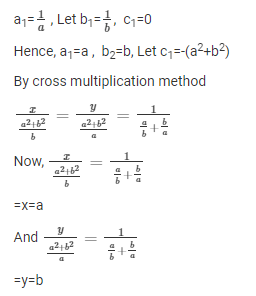Hence, the solution for the given system of equations is x = a and y = b.